# Minimum Spanning Tree n Input weighted graph GV

• Slides: 5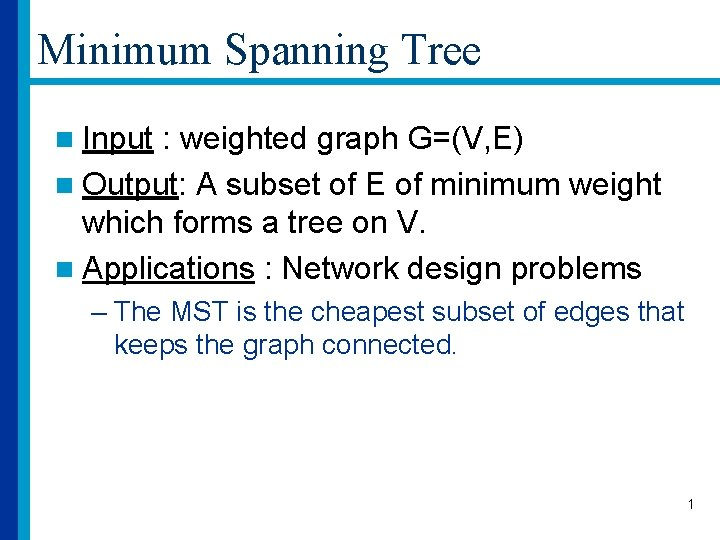Minimum Spanning Tree n Input : weighted graph G=(V, E) n Output: A subset of E of minimum weight which forms a tree on V. n Applications : Network design problems – The MST is the cheapest subset of edges that keeps the graph connected. 1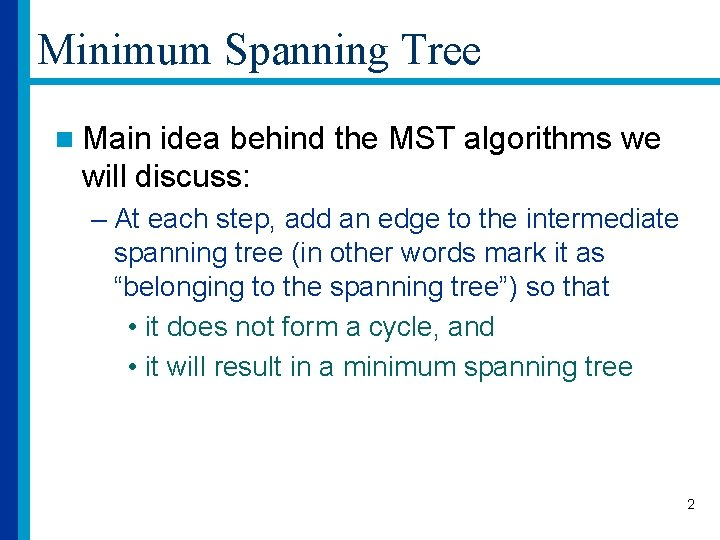Minimum Spanning Tree n Main idea behind the MST algorithms we will discuss: – At each step, add an edge to the intermediate spanning tree (in other words mark it as “belonging to the spanning tree”) so that • it does not form a cycle, and • it will result in a minimum spanning tree 2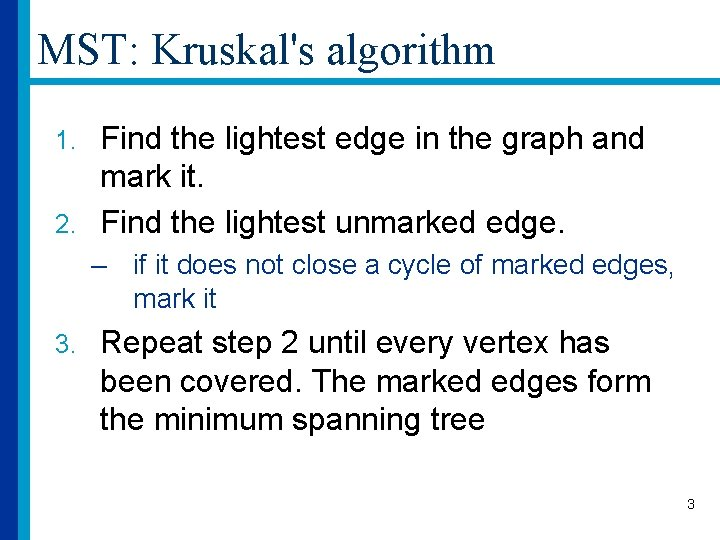MST: Kruskal's algorithm Find the lightest edge in the graph and mark it. 2. Find the lightest unmarked edge. 1. – if it does not close a cycle of marked edges, mark it 3. Repeat step 2 until every vertex has been covered. The marked edges form the minimum spanning tree 3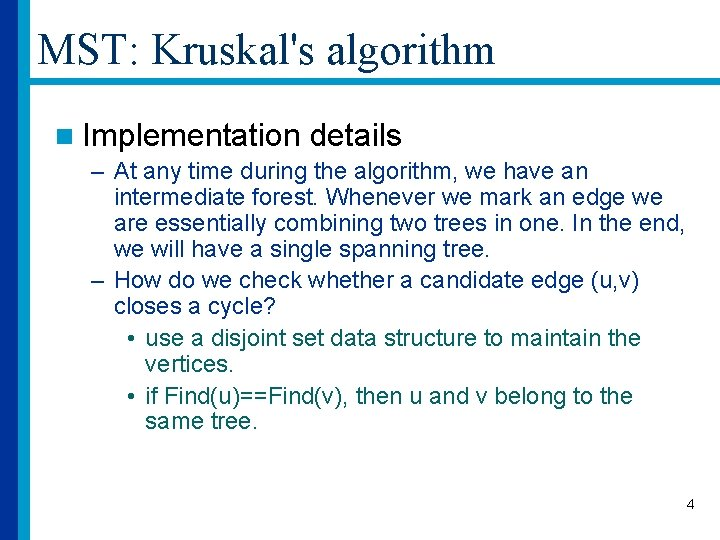MST: Kruskal's algorithm n Implementation details – At any time during the algorithm, we have an intermediate forest. Whenever we mark an edge we are essentially combining two trees in one. In the end, we will have a single spanning tree. – How do we check whether a candidate edge (u, v) closes a cycle? • use a disjoint set data structure to maintain the vertices. • if Find(u)==Find(v), then u and v belong to the same tree. 4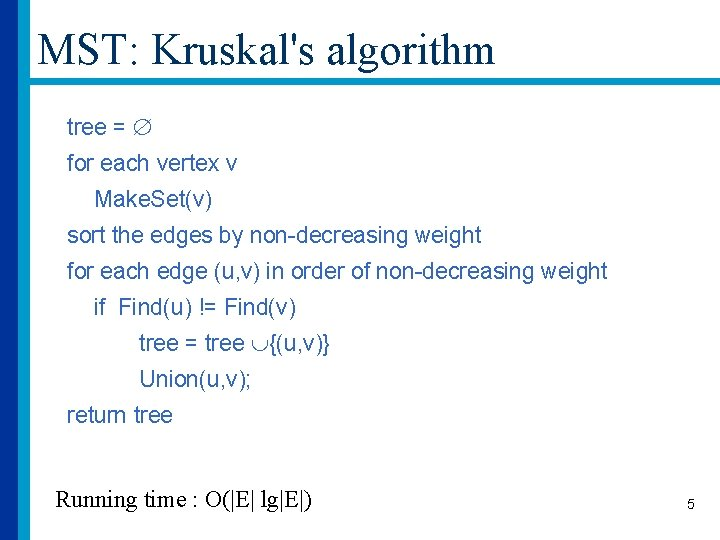MST: Kruskal's algorithm tree = for each vertex v Make. Set(v) sort the edges by non-decreasing weight for each edge (u, v) in order of non-decreasing weight if Find(u) != Find(v) tree = tree {(u, v)} Union(u, v); return tree Running time : O(|E| lg|E|) 5